## 为什么网页设计要使用栅格化 转

Yahoo! 950

MySpace 960

Live Search 958

AOL 960

960可以分解为2的6次方乘以3和5, 这使得960可以分割成以下宽度的整数倍：

2, 3, 4, 5, 6, 8, 10, 12, 15, 16, 20, 24, 30, 32, 40,
48, 60, 64, 80, 96, 120, 160, 192, 240, 320, 480

N(960) = N(2^6 * 3 * 5) = 26

N(360) = N(2^3 * 3^2 * 5) = 22
N(480) = N(2^5 * 3 * 5) = 22
N(720) = N(2^4 * 3^2 * 5) = 28
N(750) = N(2 * 3 * 5^3) = 14
N(800) = N(2^5 * 5^2) = 16
N(960) = N(2^6 * 3 * 5) = 26
N(1000) = N(2^3 * 5^3) = 14
N(1024) = N(2^10) = 9
N(1440) = N(2^6 * 3^2 * 5) = 34
N(1920) = N(2^7 * 3 * 5) = 30

A系列： …, 320, 720, 1440, …
B系列： …, 480, 960, 1920, …

N越大，可组合的宽度值就越多。对栅格系统来说，这意味着越灵活！

#page { width: 70em;}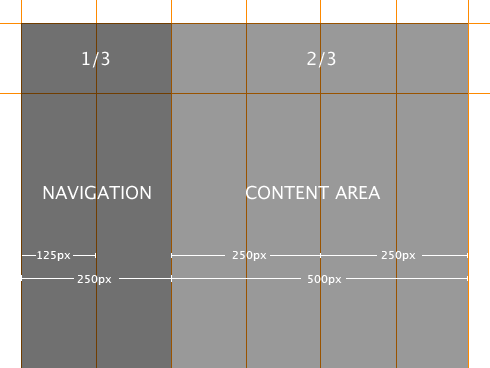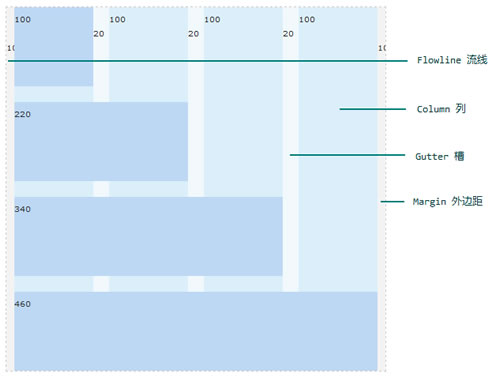W = c * N + g * (N - 1) + 2 * m

W = c * N + g * (N - 1) + g = (c + g) * N

W = C * N

950的来历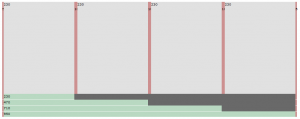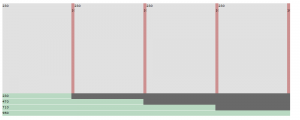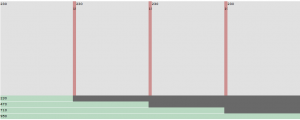W = N * C - g

950 = 12 * 80 - 10
950 = 16 * 60 - 10
950 = 24 * 40 - 10

12 x 80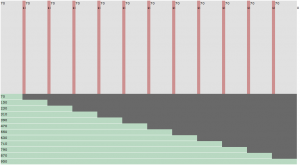16 x 60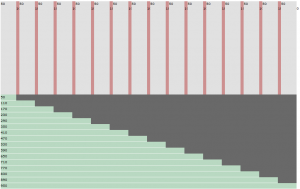24 x 40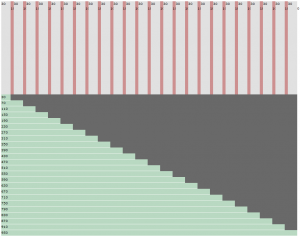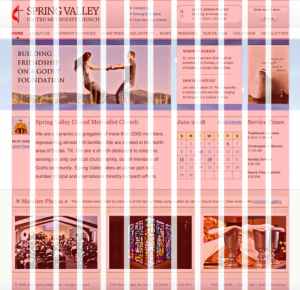Yahoo!是很标准的 24 x 40 栅格：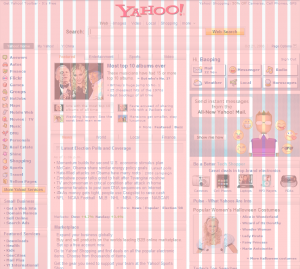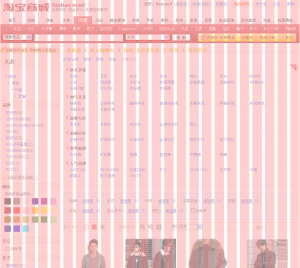x / 1 = (1 - x) / x

x^2 + x - 1 = 0

x = (sqrt(5) - 1) / 2 ~= 0.618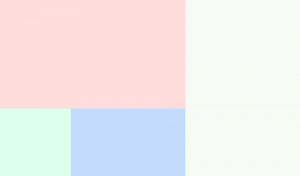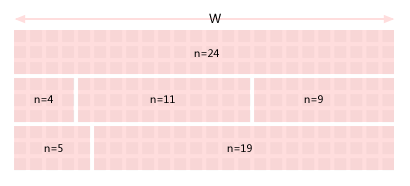N(560) = N(2^4 * 5 * 7) = 18
560 / 960 ~= 0.583
N(560)比较大，同时可以让高宽比接近黄金分割。针对560, 我们采用 14 x 40 栅格：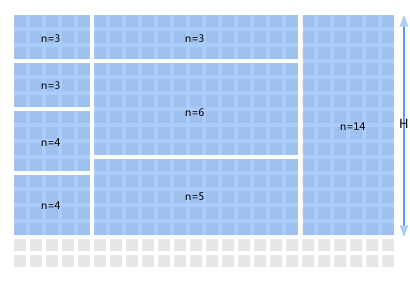（图1）

（图2）

（图3）

（图4）